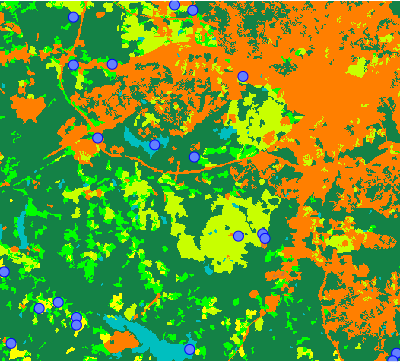## NAME

r.sample.category - Create sampling points from each category in a raster map

## KEYWORDS

raster, sampling, random, points, vector, stratified random sampling, category

## SYNOPSIS

r.sample.category
r.sample.category --help
r.sample.category [-s] input=name output=name [sampled=name[,name,...]] npoints=integer[,integer,...] [random_seed=integer] [--overwrite] [--help] [--verbose] [--quiet] [--ui]

### Flags:

-s
If number of cells in category < npoints, skip category
--overwrite
Allow output files to overwrite existing files
--help
Print usage summary
--verbose
Verbose module output
--quiet
Quiet module output
--ui
Force launching GUI dialog

### Parameters:

input=name [required]
Name of input raster map with categories (classes)
output=name [required]
Name of output vector map with points at random locations
sampled=name[,name,...]
Names of input raster maps to be sampled
npoints=integer[,integer,...] [required]
Number of sampling points per category in the input map
You can provide multiple numbers, one for each category in input raster (sorted ascending)
random_seed=integer
Seed for random number generator

## DESCRIPTION

r.sample.category generates points at random locations. Each category (class) in a raster map will contain specified number of random points.

Different number of points can be specified for different categories. For example, if there are categories 1, 4, 7 in the input raster map, and npoints=100,200,300, 100 points will be generated in category 1, 200 points in category 4 and 300 points in category 7. If only one number is specified, it will be used for every category.

## NOTES

Mask (r.mask) to create points in areas with each category, thus mask cannot be active when the module is used.

Categories are identified based on current computational region.

## EXAMPLE

### Generate random points

Generate three points at random location for each category (class) in the raster map:
```g.region raster=landclass96
r.sample.category input=landclass96 output=landclass_points npoints=3
```
Show the result:
```d.rast map=landclass96
d.vect map=landclass_points icon=basic/circle fill_color=aqua color=blue size=10
```Figure: Three random points in each category of landclass raster map

### Create a table with values sampled from rasters

Create 2 random points per each category (class) in landclass96 raster and sample elevation and geology_30m rasters at these points:
```r.sample.category input=landclass96 output=landclass_points sampled=elevation,geology_30m npoints=2
```
Look at the created data:
```v.db.select landclass_points sep=comma
```
The result of v.db.select is CSV table which can be used, for example in a spreadsheet application:
```cat,landclass96,elevation,geology_30m
1,1,102.7855,270
2,1,105.78,270
3,2,114.5954,217
4,2,137.4816,921
5,3,71.19167,270
6,3,93.33904,270
7,4,76.41077,262
8,4,97.54424,217
9,5,138.455,405
10,5,88.8075,270
11,6,126.5298,217
12,6,86.73177,217
13,7,134.5381,217
14,7,99.6844,270
```

v.sample, r.random, r.random.cells, v.random, v.what.rast, r.describe

## AUTHORS

Vaclav Petras, NCSU OSGeoREL,
Anna Petrasova, NCSU OSGeoREL

## SOURCE CODE

Available at: r.sample.category source code (history)

© 2003-2021 GRASS Development Team, GRASS GIS 7.8.7dev Reference Manual